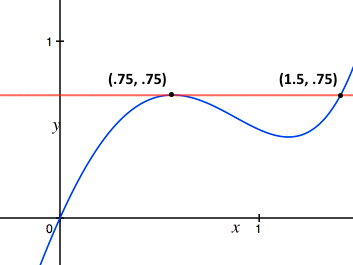Calculus

# Calculus - Basic Warmup

$\Large \frac{1}{2^0}, \frac{1}{2^1}, \frac{1}{2^2}, \frac{1}{2^3}, \frac{1}{2^4}, \ldots, \frac{1}{2^n}, \ldots$

True or False?

The sequence above approaches 0.True or False?

The slope of the red line is 0.

Police officers Alfred and Brady are stationed two kilometers apart on a road where the speed limit is 100 km/hr.

Alfred notices a car pass his position at 80 km/hr. One minute later, police officer Brady notices the same car pass his position at 80 km/hr.

Was this car driving over the speed limit as it drove along the road?

$\lim_{x \rightarrow 1 } \frac{ x^2 - 1 } { x - 1 }$

Which of the following statement is true of the above limit?

True or False?

The following infinite sum of integer reciprocals converges:

$\frac{1}{1} + \frac{1}{2} + \frac{1}{3} + \frac{1}{4} + \frac{1}{5} + \frac{1}{6} + \ldots$

(Hint: Does $1+\frac{1}{2}+\frac{1}{2}+\frac{1}{2}+...$ converge?)

×